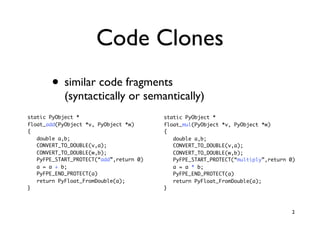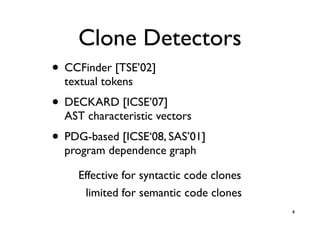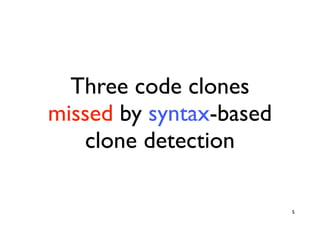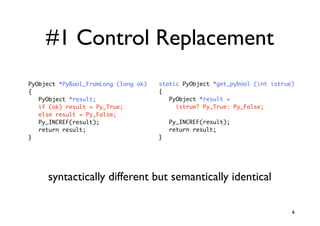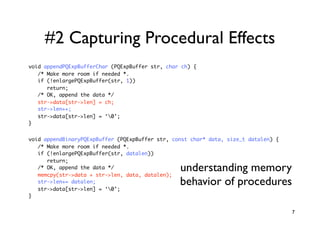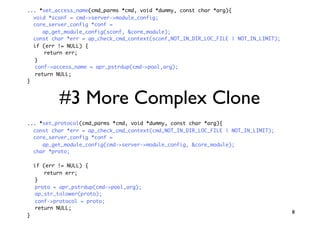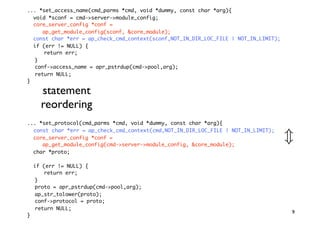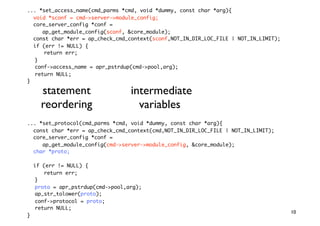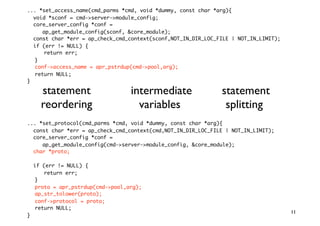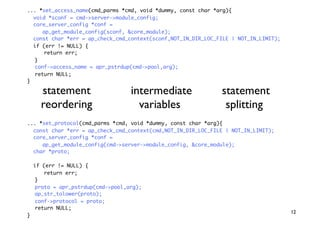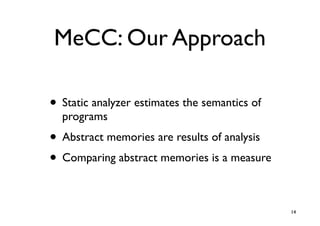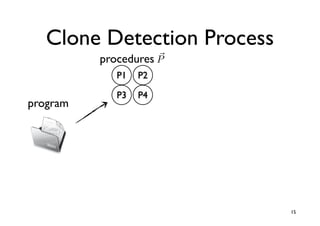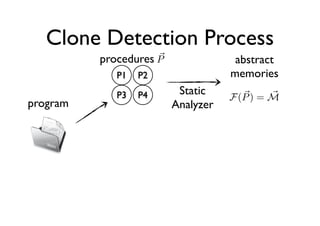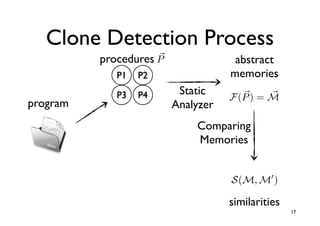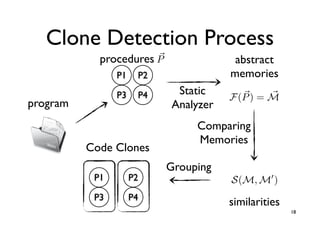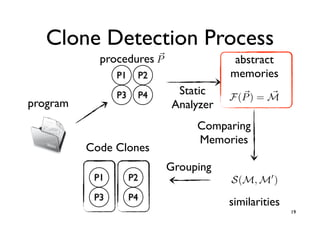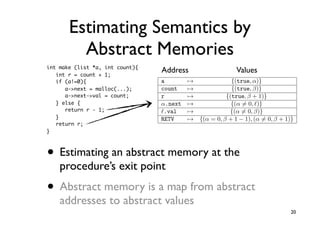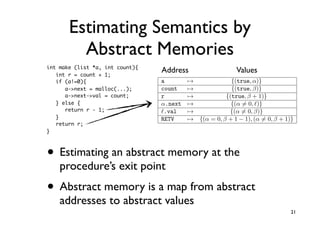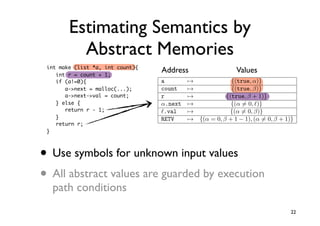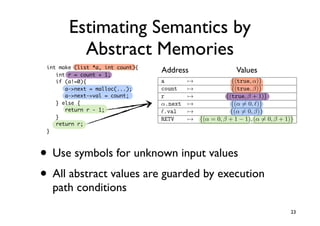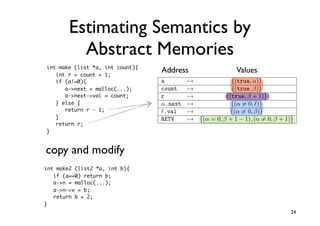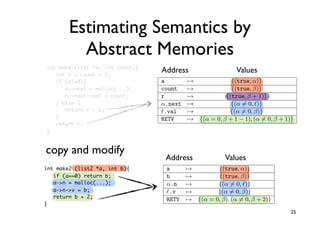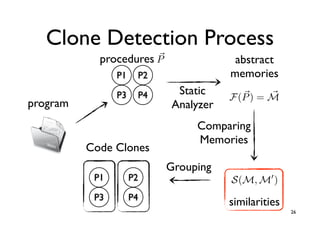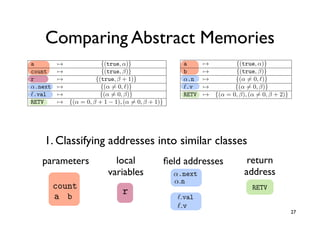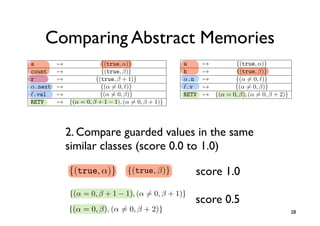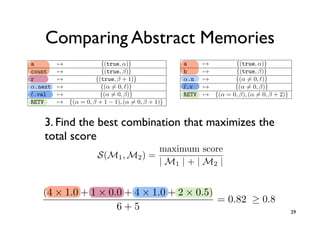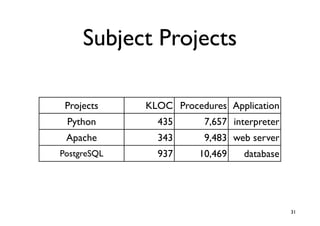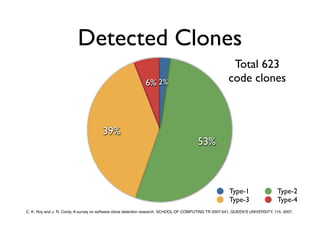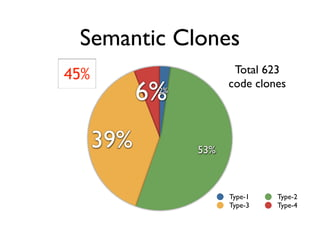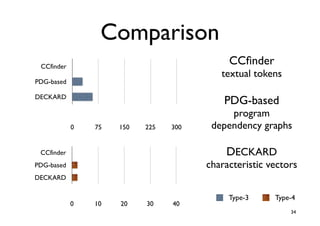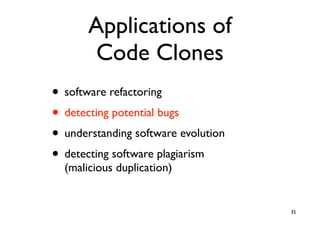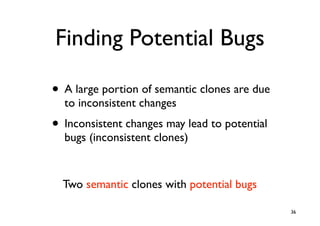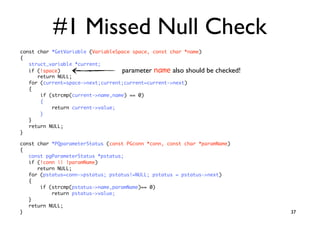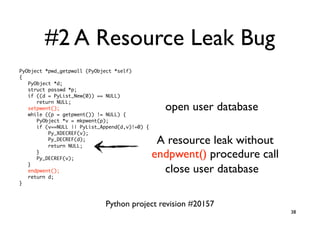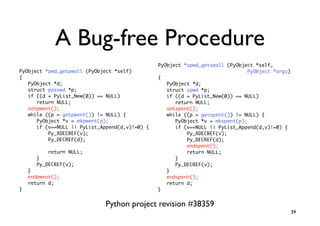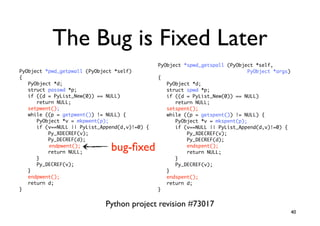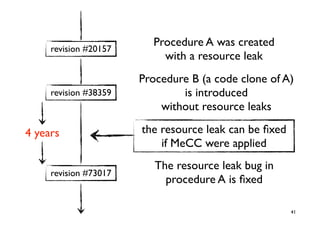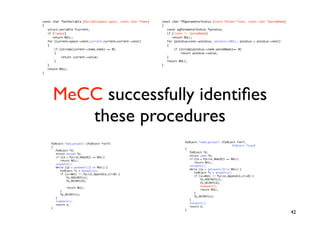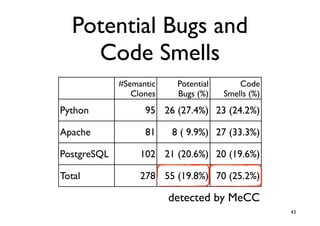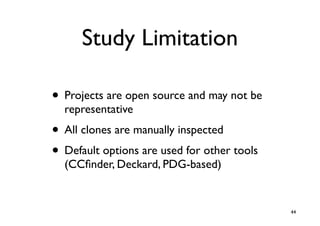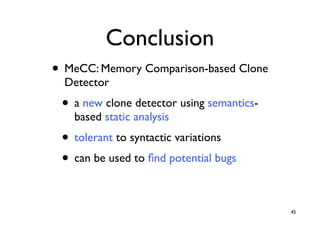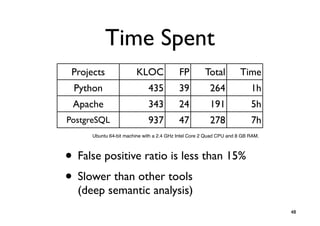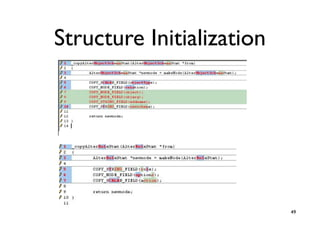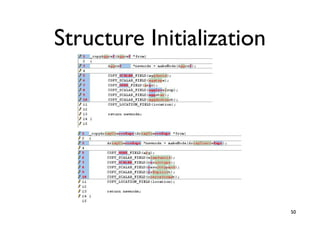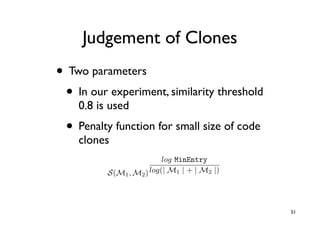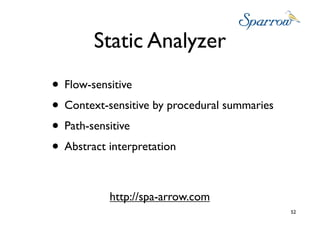1 of 52

### MeCC: Memory Comparison based Clone Detector

1. MeCC: Memory Comparison- based Clone Detector Heejung Kim1,Yungbum Jung1, Sunghun Kim2, and Kwangkeun Yi1 Seoul National University 1 2 The Hong Kong University of Science and Technology http://ropas.snu.ac.kr/mecc/ 1
2. Code Clones • similar code fragments (syntactically or semantically) static PyObject * static PyObject * float_add(PyObject *v, PyObject *w) float_mul(PyObject *v, PyObject *w) { { double a,b; double a,b; CONVERT_TO_DOUBLE(v,a); CONVERT_TO_DOUBLE(v,a); CONVERT_TO_DOUBLE(w,b); CONVERT_TO_DOUBLE(w,b); PyFPE_START_PROTECT(“add”,return 0) PyFPE_START_PROTECT(“multiply”,return 0) a = a + b; a = a * b; PyFPE_END_PROTECT(a) PyFPE_END_PROTECT(a) return PyFloat_FromDouble(a); return PyFloat_FromDouble(a); } } 2
3. Applications of Code Clones • software refactoring • detecting potential bugs • understanding software evolution • detecting software plagiarism (malicious duplication) 3
4. Clone Detectors • CCFinder [TSE’02] textual tokens • DECKARD [ICSE’07] AST characteristic vectors • PDG-based [ICSE‘08, SAS’01] program dependence graph Effective for syntactic code clones limited for semantic code clones 4
5. Three code clones missed by syntax-based clone detection 5
6. #1 Control Replacement PyObject *PyBool_FromLong (long ok) static PyObject *get_pybool (int istrue) { { PyObject *result; PyObject *result = if (ok) result = Py_True; istrue? Py_True: Py_False; else result = Py_False; Py_INCREF(result); Py_INCREF(result); return result; return result; } } syntactically different but semantically identical 6
7. #2 Capturing Procedural Effects void appendPQExpBufferChar (PQExpBuffer str, char ch) { /* Make more room if needed *. if (!enlargePQExpBuffer(str, 1)) return; /* OK, append the data */ str->data[str->len] = ch; str->len++; str->data[str->len] = ‘0’; } void appendBinaryPQExpBuffer (PQExpBuffer str, const char* data, size_t datalen) { /* Make more room if needed *. if (!enlargePQExpBuffer(str, datalen)) return; /* OK, append the data */ memcpy(str->data + str->len, data, datalen); understanding memory str->len+= datalen; str->data[str->len] = ‘0’; behavior of procedures } 7
8. ... *set_access_name(cmd_parms *cmd, void *dummy, const char *arg){ void *sconf = cmd->server->module_config; core_server_config *conf = ap_get_module_config(sconf, &core_module); const char *err = ap_check_cmd_context(sconf,NOT_IN_DIR_LOC_FILE | NOT_IN_LIMIT); if (err != NULL) { return err; } conf->access_name = apr_pstrdup(cmd->pool,arg); return NULL; } #3 More Complex Clone ... *set_protocol(cmd_parms *cmd, void *dummy, const char *arg){ const char *err = ap_check_cmd_context(cmd,NOT_IN_DIR_LOC_FILE | NOT_IN_LIMIT); core_server_config *conf = ap_get_module_config(cmd->server->module_config, &core_module); char *proto; if (err != NULL) { return err; } proto = apr_pstrdup(cmd->pool,arg); ap_str_tolower(proto); conf->protocol = proto; return NULL; 8 }
9. ... *set_access_name(cmd_parms *cmd, void *dummy, const char *arg){ void *sconf = cmd->server->module_config; core_server_config *conf = ap_get_module_config(sconf, &core_module); const char *err = ap_check_cmd_context(sconf,NOT_IN_DIR_LOC_FILE | NOT_IN_LIMIT); if (err != NULL) { return err; } conf->access_name = apr_pstrdup(cmd->pool,arg); return NULL; } statement reordering ... *set_protocol(cmd_parms *cmd, void *dummy, const char *arg){ const char *err = ap_check_cmd_context(cmd,NOT_IN_DIR_LOC_FILE | NOT_IN_LIMIT); core_server_config *conf = ap_get_module_config(cmd->server->module_config, &core_module); char *proto; if (err != NULL) { return err; } proto = apr_pstrdup(cmd-pool,arg); ap_str_tolower(proto); conf-protocol = proto; return NULL; 9 }
10. ... *set_access_name(cmd_parms *cmd, void *dummy, const char *arg){ void *sconf = cmd-server-module_config; core_server_config *conf = ap_get_module_config(sconf, core_module); const char *err = ap_check_cmd_context(sconf,NOT_IN_DIR_LOC_FILE | NOT_IN_LIMIT); if (err != NULL) { return err; } conf-access_name = apr_pstrdup(cmd-pool,arg); return NULL; } statement intermediate reordering variables ... *set_protocol(cmd_parms *cmd, void *dummy, const char *arg){ const char *err = ap_check_cmd_context(cmd,NOT_IN_DIR_LOC_FILE | NOT_IN_LIMIT); core_server_config *conf = ap_get_module_config(cmd-server-module_config, core_module); char *proto; if (err != NULL) { return err; } proto = apr_pstrdup(cmd-pool,arg); ap_str_tolower(proto); conf-protocol = proto; return NULL; 10 }
11. ... *set_access_name(cmd_parms *cmd, void *dummy, const char *arg){ void *sconf = cmd-server-module_config; core_server_config *conf = ap_get_module_config(sconf, core_module); const char *err = ap_check_cmd_context(sconf,NOT_IN_DIR_LOC_FILE | NOT_IN_LIMIT); if (err != NULL) { return err; } conf-access_name = apr_pstrdup(cmd-pool,arg); return NULL; } statement intermediate statement reordering variables splitting ... *set_protocol(cmd_parms *cmd, void *dummy, const char *arg){ const char *err = ap_check_cmd_context(cmd,NOT_IN_DIR_LOC_FILE | NOT_IN_LIMIT); core_server_config *conf = ap_get_module_config(cmd-server-module_config, core_module); char *proto; if (err != NULL) { return err; } proto = apr_pstrdup(cmd-pool,arg); ap_str_tolower(proto); conf-protocol = proto; return NULL; 11 }
12. ... *set_access_name(cmd_parms *cmd, void *dummy, const char *arg){ void *sconf = cmd-server-module_config; core_server_config *conf = ap_get_module_config(sconf, core_module); const char *err = ap_check_cmd_context(sconf,NOT_IN_DIR_LOC_FILE | NOT_IN_LIMIT); if (err != NULL) { return err; } conf-access_name = apr_pstrdup(cmd-pool,arg); return NULL; } statement intermediate statement reordering variables splitting ... *set_protocol(cmd_parms *cmd, void *dummy, const char *arg){ const char *err = ap_check_cmd_context(cmd,NOT_IN_DIR_LOC_FILE | NOT_IN_LIMIT); core_server_config *conf = ap_get_module_config(cmd-server-module_config, core_module); char *proto; if (err != NULL) { return err; } proto = apr_pstrdup(cmd-pool,arg); ap_str_tolower(proto); conf-protocol = proto; return NULL; 12 }
13. These Semantic Clones are Identiﬁed by MeCC 13
14. MeCC: Our Approach • Static analyzer estimates the semantics of programs • Abstract memories are results of analysis • Comparing abstract memories is a measure 14
15. Clone Detection Process procedures P P1 P2 P3 P4 program 15
16. Clone Detection Process procedures P abstract P1 P2 memories P3 P4 Static F (P ) = M program Analyzer
17. Clone Detection Process procedures P abstract P1 P2 memories P3 P4 Static F (P ) = M program Analyzer Comparing Memories S(M, M ) similarities 17
18. Clone Detection Process procedures P abstract P1 P2 memories P3 P4 Static F (P ) = M program Analyzer Comparing Memories Code Clones Grouping P1 P2 S(M, M ) P3 P4 similarities 18
19. Clone Detection Process procedures P abstract P1 P2 memories P3 P4 Static F (P ) = M program Analyzer Comparing Memories Code Clones Grouping P1 P2 S(M, M ) P3 P4 similarities 19
20. Estimating Semantics by log MinEntry Abstract Memories S(M1 , M2 ) log(| M1 | + | M2 |) 2(2 · 1.0 + 2 · 1.0 + 1 · 0.5)/(6 + 5) = 0.82 int make (list *a, int count){ int r = count + 1; Address Values if (a!=0){ a → {(true, α)} a-next = malloc(...); count → {(true, β)} a-next-val = count; r → {(true, β + 1)} } else { α.next → {(α = 0, )} return r - 1; .val → {(α = 0, β)} } RETV → {(α = 0, β + 1 − 1), (α = 0, β + 1)} return r; } a → {(true, α)} b → {(true, β)} • Estimating an abstract memory at the α.n .v → → {(α = 0, )} {(α = 0, β)} procedure’s exit point RETV → {(α = 0, β), (α = 0, β + 2)} {}, {} P ⇓ v, M • Abstract memory is a map from abstract {}, {} P : τ addresses to abstractlist next} type list = {int x, values 20 let list node = {x:=1, next:={}}
21. Estimating Semantics by log MinEntry Abstract Memories S(M1 , M2 ) log(| M1 | + | M2 |) 2(2 · 1.0 + 2 · 1.0 + 1 · 0.5)/(6 + 5) = 0.82 int make (list *a, int count){ int r = count + 1; Address Values if (a!=0){ a → {(true, α)} a-next = malloc(...); count → {(true, β)} a-next-val = count; r → {(true, β + 1)} } else { α.next → {(α = 0, )} return r - 1; .val → {(α = 0, β)} } RETV → {(α = 0, β + 1 − 1), (α = 0, β + 1)} return r; } a → {(true, α)} b → {(true, β)} • Estimating an abstract memory at the α.n .v → → {(α = 0, )} {(α = 0, β)} procedure’s exit point RETV → {(α = 0, β), (α = 0, β + 2)} {}, {} P ⇓ v, M • Abstract memory is a map from abstract {}, {} P : τ addresses to abstractlist next} type list = {int x, values 21 let list node = {x:=1, next:={}}
22. Estimating Semantics by log MinEntry Abstract Memories S(M1 , M2 ) log(| M1 | + | M2 |) 2(2 · 1.0 + 2 · 1.0 + 1 · 0.5)/(6 + 5) = 0.82 int make (list *a, int count){ int r = count + 1; Address Values if (a!=0){ a → {(true, α)} a-next = malloc(...); count → {(true, β)} a-next-val = count; r → {(true, β + 1)} } else { α.next → {(α = 0, )} return r - 1; .val → {(α = 0, β)} } RETV → {(α = 0, β + 1 − 1), (α = 0, β + 1)} return r; } a → {(true, α)} b → {(true, β)} • Use symbols for unknown input values α.n → {(α = 0, )} .v → {(α = 0, β)} RETV → {(α = 0, β), (α = 0, β + 2)} • All abstract values are guarded by execution {}, {} P ⇓ v, M path conditions {}, {} P : τ type list = {int x, list next} 22 let list node = {x:=1, next:={}}
23. Estimating Semantics by log MinEntry Abstract Memories S(M1 , M2 ) log(| M1 | + | M2 |) 2(2 · 1.0 + 2 · 1.0 + 1 · 0.5)/(6 + 5) = 0.82 int make (list *a, int count){ int r = count + 1; Address Values if (a!=0){ a → {(true, α)} a-next = malloc(...); count → {(true, β)} a-next-val = count; r → {(true, β + 1)} } else { α.next → {(α = 0, )} return r - 1; .val → {(α = 0, β)} } RETV → {(α = 0, β + 1 − 1), (α = 0, β + 1)} return r; } a → {(true, α)} b → {(true, β)} • Use symbols for unknown input values α.n → {(α = 0, )} .v → {(α = 0, β)} RETV → {(α = 0, β), (α = 0, β + 2)} • All abstract values are guarded by execution {}, {} P ⇓ v, M path conditions {}, {} P : τ type list = {int x, list next} 23 let list node = {x:=1, next:={}}
24. Estimating Semantics by log MinEntry Abstract Memories S(M1 , M2 ) log(| M1 | + | M2 |) 2(2 · 1.0 + 2 · 1.0 + 1 · 0.5)/(6 + 5) = 0.82 int make (list *a, int count){ int r = count + 1; Address Values if (a!=0){ a → {(true, α)} a-next = malloc(...); count → {(true, β)} a-next-val = count; r → {(true, β + 1)} } else { α.next → {(α = 0, )} return r - 1; .val → {(α = 0, β)} } RETV → {(α = 0, β + 1 − 1), (α = 0, β + 1)} return r; } a → {(true, α)} b → {(true, β)} copy and modify α.n .v → → {(α = 0, )} {(α = 0, β)} RETV → {(α = 0, β), (α = 0, β + 2)} int make2 (list2 *a, int b){ if (a==0) return b; {}, {} P ⇓ v, M a-n = malloc(...); a-n-v = b; return b + 2; {}, {} P : τ } type list = {int x, list next} 24 let list node = {x:=1, next:={}}
25. Estimating Semantics by log MinEntry Abstract Memories S(M1 , M2 ) log(| M1 | + | M2 |) 2(2 · 1.0 + 2 · 1.0 + 1 · 0.5)/(6 + 5) = 0.82 int make (list *a, int count){ int r = count + 1; Address Values log MinEntry if (a!=0){ a S(M→M ) log(| M1 {(true, α)} , 1 2 | + | M2 |) a-next = malloc(...); count → {(true, β)} a-next-val = count; r → {(true, β + 1)} } else { 2(2 · 1.0 + 2 · 1.0 + 1 · 0.5)/(6 + 5) = 0.82 α.next → {(α = 0, )} return r - 1; .val → {(α = 0, β)} } a RETV → → {(α = 0, {(true, α)}(α = 0, β + 1)} β + 1 − 1), return r; count → {(true, β)} } r a→ → {(true, 1)} {(true, β +α)} α.next b→ → {(true, β)} {(α = 0, )} copy and modify .val α.n →→ {(α = = 0, )} {(α 0, β)} RETV Address → = 0, β + Values(α = 0, β + 1)} .v {(α → {(α = 1 − 1), 0, β)} RETV → {(α = 0, β), (α = 0, β + 2)} int make2 (list2 *a, int b){ a → {(true, α)} if (a==0) return b; b → {}, {} {(true, β)} P ⇓ v, M a-n = malloc(...); α.n → {(α = 0, )} a-n-v = b; → return b + 2; .v {}, {(α = 0, τ {} P : β)} RETV → {(α = 0, β), (α = 0, β + 2)} } type list = {int x, list next} {}, {} P ⇓ v, M 25 let list node = {x:=1, next:={}}
26. Clone Detection Process procedures P abstract P1 P2 memories P3 P4 Static F (P ) = M program Analyzer Comparing Memories Code Clones Grouping P1 P2 S(M, M ) P3 P4 similarities 26
27. a → {(true, α)} log MinEntry count → {(true, β)} S(M1 , M2 ) log(| M1 | + | M2 |) r → {(true, β + 1)} Comparing Abstract Memories 2(2 · 1.0 + 2 · 1.0 + 1 · 0.5)/(6 + 5) = 0.82 α.next .val → → {(α = 0, )} {(α = 0, β)} RETV → {(α = 0, β + 1 − 1), (α = 0, β + 1)} a → {(true, α)} a → {(true, α)} count → {(true, β)} b → {(true, β)} r → {(true, β + 1)} α.n → {(α = 0, )} α.next → {(α = 0, )} .v → {(α = 0, β)} .val → {(α = 0, β)} RETV a {(tru → {(α = 0, β), (α = 0, β + 2)} RETV → {(α = 0, β + 1 − 1), (α = 0, β + 1)} count {(tru {}, {} P ⇓ v, M aa → {(true, α)} {(true, α)} r {(true, b → {(true, β)} α.next {(α = count α.n → {(α = 0, )} {(true,log MinEntry β)} {}, {} P : τ α.val {(α = r → {(α = 0, β)} M2 ) log(| M1 | + | M2 |) {(true, β + 1)} 1. Classifying addresses into similar classes .v α.next S(M1 , type list = {int x, list next} MinEntry RETV a log RETV → {(α = 0, β), (α = 0, {(α2)} 0, )} log MinEntry β + = {(true, α)} {(α = 0, β + 1 − 1 S(M , M log(| M1 | + | M2 |) a {(true α.val {}, {} 2(2letM S(M1.0 +21)1·log(| )M+ |5) = M2 |) {(true, β)} {(α ,= {x:=1,2 next:={}} 0.82 list node =0, β)} count + | ·v, local return parameters P ⇓in1.0 + 2 · 1 M ﬁeld addresses {(true, β + 1)} {(true 0.5)/(6 1 r 0, β + 1)} {(true, α)} b RETV {(α = 0, βa 1 − 1), (α = + a node.next.x 2(2{(true, α)} α.next address variables· 1.0 + 2 · 1.0 + 1 · 0.5)/(6 + 5) ={(αα.n0, )} = 0.82β)} {(α = 0 {}, {} P : τ count .val 1.0 + 1α.n .v α.val {(true,α.v β)} +{(true, in0.5)/(6 + 5) = 0.82 = 0, 2(2x· 1.0 {a:=1,α)} β)}E 2 · b:=2} · {(α {(α = 0 count a let {(true, := = {int x, list next} a b a r RETV {(true, .val α)} {(true, β)}βlist1)} .v {(true, βRETV (α{(α = 0, β), (α {(α = 0, β + 1 −+ 1)} 0, β + 1)} 1), = r type list{(true, {(true, α)} → α.next x, = {int + next} {(α {(α )}0, .vprev} 0, x, tsil α.n .val β)} α.nextcount type tsil =={(true,)} β)} ode = {x:=1, next:={}} countα.n {int = {(true, a {(α {(true,)} {}, {} P ⇓ v = 0, α)} → 27 → = 0, β b {(true, α)} = 0, β)} α.val {(true, a{(α = 0, β)} β)} + 1)} {(α {(true, β)} xt.x r α.v α.val r let→ {(true, β + {(α ... {x:=1, next:={}} 1)}
28. a → {(true, α)} log MinEntry count → {(true, β)} S(M1 , M2 ) log(| M1 | + | M2 |) r → {(true, β + 1)} Comparing Abstract Memories 2(2 · 1.0 + 2 · 1.0 + 1 · 0.5)/(6 + 5) = 0.82 α.next .val → → {(α = 0, )} {(α = 0, β)} RETV → {(α = 0, β + 1 − 1), (α = 0, β + 1)} a → {(true, α)} a → {(true, α)} count → {(true, β)} b → {(true, β)} r → {(true, β + 1)} α.n → {(α = 0, )} α.next → {(α = 0, )} .v → {(α = 0, β)} .val → {(α = 0, β)} RETV → {(α = 0, β), (α = 0, β + 2)} RETV → {(α = 0, β + 1 − 1), (α = 0, β + 1)} a {(true, α)} {}, {} P ⇓ v, M counta → {(true, α)} β)} {(true, b → {(true, β)} r {(true, )}+ 1)} {(α = 0, β {}, {} P : τ α.n → α.next .v 2. Compareβ)} )} → {(α = guarded values in the same {(α = 0, 0, type list = {int x, list next} α.val similar classes (score 0.0 to 1.0) RETV → {(α = 0, {(α = 0, β + 2)} β), (α = 0, β)} RETV {(α {}, {} β P ⇓letM1), (α = 0, β + 1)} − = 0, + 1v, list node = {x:=1, next:={}} a in {(true, α)} count {(true, α)} α)} a {(true, {(true, β)} {}, {} P : τ node.next.x score 1.0 t r b {(true, β)} x{(true, β b:=2} in E {(true, β)} let := {a:=1, + 1)} α.next = 0, β+ = − )}(α= 0, )} 1)} = {int x, listα.n next} {(α 1 0, {(α {(true, β +1)} 1), = 0, β + {(α α.val{(α = {(α = 0, β)}= = 0, β)} α.v 0, )} ={(αβ + 2)} type list score {int x, list next} ext = {x:=1, next:={}}{(α = 0, β), (α tsil = {int x, tsil prev} ode 0.5 RETV RETV {(α =typeβ +0, − 1), (α = 0, β + 1)} 0, 1 28 al xt.x {(α = 0, β)} let ... {x:=1, next:={}}
29. a → {(true, α)} log MinEntry count → {(true, β)} S(M1 , M2 ) log(| M1 | + | M2 |) r → {(true, β + 1)} Comparing Abstract Memories 2(2 · 1.0 + 2 · 1.0 + 1 · 0.5)/(6 + 5) = 0.82 α.next .val → → {(α = 0, )} {(α = 0, β)} RETV → {(α = 0, β + 1 − 1), (α = 0, β + 1)} a → {(true, α)} a → {(true, α)} count → {(true, β)} b → {(true, β)} r → {(true, β + 1)} α.n → {(α = 0, )} α.next → {(α = 0, )} .v → {(α = 0, β)} .val → {(α = 0, β)} {(true, α)}RETV → {(α = 0, β), (α = 0, β + 2)} RETV → {(α = 0, β + 1 − 1), (α = 0, β + 1)} {}, {} P ⇓ v, M a → {(true, α)} →(4 × 1.0 + 1 β)} 0.0 + 4 × 1.0 + 2 × {(true, × 0.5) 3. Find the best combination that maximizes the b α.n → {}, = P : τ {} 0.82 {(α = 0, )} 6 + 5 total score .v → {(α = 0, β)} type list = {int x, list next} RETV → {(α = 0, β), (α = 0, β + 2)} maximum score {}, {} P ⇓ v, M1 , M2 ) = S(Mlist node = {x:=1, next:={}} let in {(true, α)} 1 | + | M2 | |M node.next.x {}, {} P : τ | {a:=1, − F(c )E| let x := F(c) b:=2} in (4 × 1.0 + 1 × 0.0 + 4 × 1.0 + 2 × 0.5) = {int x, list next} type list = {int x, list next} = 0.82 ≥ 0.8 ode = {x:=1, next:={}} type6tsil 5 {int x, tsil prev} + = 29 10 xt.x let ... {x:=1, next:={}}
30. Experimental Results 30
31. Subject Projects Projects KLOC Procedures Application Python 435 7,657 interpreter Apache 343 9,483 web server PostgreSQL 937 10,469 database 31
32. Detected Clones Total 623 6% 2% code clones 39% 53% Type-1 Type-2 Type-3 Type-4 C. K. Roy and J. R. Cordy. A survey on software clone detection research. SCHOOL OF COMPUTING TR 2007-541, QUEENʼS UNIVERSITY, 115, 2007.
33. Semantic Clones 45% Total 623 6% 2% code clones 39% 53% Type-1 Type-2 Type-3 Type-4
34. Comparison CCfinder CCﬁnder textual tokens PDG-based DECKARD PDG-based MeCC program 0 75 150 225 300 dependency graphs CCfinder DECKARD PDG-based characteristic vectors DECKARD MeCC Type-3 Type-4 0 10 20 30 40 34
35. Applications of Code Clones • software refactoring • detecting potential bugs • understanding software evolution • detecting software plagiarism (malicious duplication) 35
36. Finding Potential Bugs • A large portion of semantic clones are due to inconsistent changes • Inconsistent changes may lead to potential bugs (inconsistent clones) Two semantic clones with potential bugs 36
37. #1 Missed Null Check const char *GetVariable (VariableSpace space, const char *name) { struct_variable *current; if (!space) parameter name also should be checked! return NULL; for (current=space-next;current;current=current-next) { if (strcmp(current-name,name) == 0) { return current-value; } } return NULL; } const char *PQparameterStatus (const PGconn *conn, const char *paramName) { const pgParameterStatus *pstatus; if (!conn || !paramName) return NULL; for (pstatus=conn-pstatus; pstatus!=NULL; pstatus = pstatus-next) { if (strcmp(pstatus-name,paramName)== 0) return pstatus-value; } return NULL; } 37
38. #2 A Resource Leak Bug PyObject *pwd_getpwall (PyObject *self) { PyObject *d; struct passwd *p; if ((d = PyList_New(0)) == NULL) return NULL; setpwent(); open user database while ((p = getpwent()) != NULL) { PyObject *v = mkpwent(p); if (v==NULL || PyList_Append(d,v)!=0) { Py_XDECREF(v); Py_DECREF(d); return NULL; A resource leak without } Py_DECREF(v); endpwent() procedure call } endpwent(); close user database return d; } Python project revision #20157 38
39. A Bug-free Procedure PyObject *spwd_getspall (PyObject *self, PyObject *pwd_getpwall (PyObject *self) PyObject *args) { { PyObject *d; PyObject *d; struct passwd *p; struct spwd *p; if ((d = PyList_New(0)) == NULL) if ((d = PyList_New(0)) == NULL) return NULL; return NULL; setpwent(); setspent(); while ((p = getpwent()) != NULL) { while ((p = getspent()) != NULL) { PyObject *v = mkpwent(p); PyObject *v = mkspent(p); if (v==NULL || PyList_Append(d,v)!=0) { if (v==NULL || PyList_Append(d,v)!=0) { Py_XDECREF(v); Py_XDECREF(v); Py_DECREF(d); Py_DECREF(d); endspent(); return NULL; return NULL; } } Py_DECREF(v); Py_DECREF(v); } } endpwent(); endspent(); return d; return d; } } Python project revision #38359 39
40. The Bug is Fixed Later PyObject *spwd_getspall (PyObject *self, PyObject *pwd_getpwall (PyObject *self) PyObject *args) { { PyObject *d; PyObject *d; struct passwd *p; struct spwd *p; if ((d = PyList_New(0)) == NULL) if ((d = PyList_New(0)) == NULL) return NULL; return NULL; setpwent(); setspent(); while ((p = getpwent()) != NULL) { while ((p = getspent()) != NULL) { PyObject *v = mkpwent(p); PyObject *v = mkspent(p); if (v==NULL || PyList_Append(d,v)!=0) { if (v==NULL || PyList_Append(d,v)!=0) { Py_XDECREF(v); Py_XDECREF(v); Py_DECREF(d); Py_DECREF(d); endpwent(); return NULL; bug-ﬁxed endspent(); return NULL; } } Py_DECREF(v); Py_DECREF(v); } } endpwent(); endspent(); return d; return d; } } Python project revision #73017 40
41. Procedure A was created revision #20157 with a resource leak Procedure B (a code clone of A) revision #38359 is introduced without resource leaks 4 years the resource leak can be ﬁxed if MeCC were applied The resource leak bug in revision #73017 procedure A is ﬁxed 41
42. const char *GetVariable (VariableSpace space, const char *name) const char *PQparameterStatus (const PGconn *conn, const char *paramName) { { struct_variable *current; const pgParameterStatus *pstatus; if (!space) if (!conn || !paramName) return NULL; return NULL; for (current=space-next;current;current=current-next) for (pstatus=conn-pstatus; pstatus!=NULL; pstatus = pstatus-next) { { if (strcmp(current-name,name) == 0) if (strcmp(pstatus-name.paramName)== 0) { return pstatus-value; return current-value; } } return NULL; } } return NULL; } MeCC successfully identiﬁes these procedures PyObject *spwd_getspall (PyObject *self, PyObject *pwd_getpwall (PyObject *self) PyObject *args) { { PyObject *d; PyObject *d; struct passwd *p; struct spwd *p; if ((d = PyList_New(0)) == NULL) if ((d = PyList_New(0)) == NULL) return NULL; return NULL; setpwent(); setspent(); while ((p = getpwent()) != NULL) { while ((p = getspent()) != NULL) { PyObject *v = mkpwent(p); PyObject *v = mkspent(p); if (v==NULL || PyList_Append(d,v)!=0) { if (v==NULL || PyList_Append(d,v)!=0) { Py_XDECREF(v); Py_XDECREF(v); Py_DECREF(d); Py_DECREF(d); endspent(); return NULL; return NULL; } } Py_DECREF(v); Py_DECREF(v); } } endpwent(); endspent(); return d; return d; } } 42
43. Potential Bugs and Code Smells #Semantic Potential Code Clones Bugs (%) Smells (%) Python 95 26 (27.4%) 23 (24.2%) Apache 81 8 ( 9.9%) 27 (33.3%) PostgreSQL 102 21 (20.6%) 20 (19.6%) Total 278 55 (19.8%) 70 (25.2%) detected by MeCC 43
44. Study Limitation • Projects are open source and may not be representative • All clones are manually inspected • Default options are used for other tools (CCﬁnder, Deckard, PDG-based) 44
45. Conclusion • MeCC: Memory Comparison-based Clone Detector • a new clone detector using semantics- based static analysis • tolerant to syntactic variations • can be used to ﬁnd potential bugs 45
46. Thank You! http://ropas.snu.ac.kr/mecc/ 46
47. Backup Slides 47
48. Time Spent Projects KLOC FP Total Time Python 435 39 264 1h Apache 343 24 191 5h PostgreSQL 937 47 278 7h Ubuntu 64-bit machine with a 2.4 GHz Intel Core 2 Quad CPU and 8 GB RAM. • False positive ratio is less than 15% • Slower than other tools (deep semantic analysis) 48
49. Structure Initialization 49
50. Structure Initialization 50
51. Judgement of Clones • Two parameters • In our experiment, similarity threshold 0.8 is used • Penalty function for small size of code clones log MinEntry S(M1 , M2 ) log(| M1 | + | M2 |) 2(2 · 1.0 + 2 · 1.0 + 1 · 0.5)/(6 + 5) = 0.82 51 a {(true, α)}
52. Static Analyzer • Flow-sensitive • Context-sensitive by procedural summaries • Path-sensitive • Abstract interpretation http://spa-arrow.com 52